Processing ......FreeComputerBooks.com Links to Free Computer, Mathematics, Technical Books all over the World

General and Miscellaneous Mathematics
Related Book Categories:
•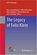The Legacy of Felix Klein (Hans-Georg Weigand, et al)

This open access book provides an overview of Felix Klein's ideas. It discusses the meaning, importance and the legacy of Klein's ideas today and in the future, within an international, global context.

•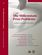The Millennium Prize Problems (James Carlson, et al)

Guided by the premise that solving some of the world's most important mathematical problems will advance the field, this book offers a fascinating look at the seven unsolved Millennium Prize problems.

•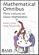Mathematical Omnibus: Thirty Lectures on Classic Mathematics

This is an enjoyable book with suggested uses ranging from a text for a undergraduate Honors Mathematics Seminar to a coffee table book. The common thread in the selected subjects is their illustration of the unity and beauty of mathematics.

•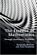The Essence of Mathematics Through Elementary Problems

This book consists of a sequence of 270 problems of increasing sophistication (Mental Skills; Arithmetic; Word Problems; Algebra; Geometry; Infinity) – with commentary and full solutions. The reader is assumed to have a reasonable grasp of school mathematics.

•Mathematics in the Age of the Turing Machine (Thomas C. Hales)

Computers have rapidly become so pervasive in mathematics that future generations may look back to this day as a golden dawn. The article gives a survey of mathematical proofs that rely on computer calculations and formal proofs.

•Math in Society: A Survey of Mathematics for the Liberal Arts Major

This book is a survey of contemporary mathematical topics, most non-algebraic, appropriate for a college-level quantitative literacy topics course for liberal arts majors. Emphasis is placed on the applicability of the mathematics.

•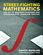Street-Fighting Math: Guessing & Opportunistic Problem Solving

This engaging book is an antidote to the rigor mortis brought on by too much mathematical rigor, teaching us how to guess answers without needing a proof or an exact calculation.

•Six Septembers: Mathematics for the Humanist (Patrick Juola)

Developing the skills to enable humanist scholars to address complicated technical material with confidence. Mathematics operates under a regime of shared assumptions, and it is our purpose to elucidate some of those assumptions for the newcomer.

•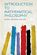Introduction to Mathematical Philosophy (Bertrand Russell)

Requiring neither prior knowledge of mathematics nor aptitude for mathematical symbolism, the book serves as essential reading for anyone interested in the intersection of mathematics and logic and in the development of analytic philosophy.

•What is Mathematics: Godel's Theorem and Around (K. Podnieks)

This accessible book gives a new, detailed and elementary explanation of the Godel incompleteness theorems and presents the Chaitin results and their relation to the da Costa-Doria results, which are given in full, but with no technicalities.

•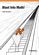Blast Into Math! (Julie Rowlett)

It is a fun and rigorous introduction to pure mathematics, is suitable for both students and a general audience interested in learning what pure mathematics is all about. Pure mathematics is presented in a friendly, accessible, and nonetheless rigorous style.

•Math Alive (Ingrid Daubechies, et at)

Mathematics has profoundly changed our world, from banking to listening to music. This course is designed for those who haven't had college mathematics but would like to understand some of the mathematical concepts behind important modern applications.

•Mathematics Illuminated (MacGregor Campbell)

This book is a 13-part, integrated-media resource created for adult learners and high school teachers. The series covers the broad scope of human knowledge through the study of mathematics and its relevance in the world today.

•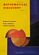Mathematical Discovery (A.M. Bruckner, et al)

A course introducing the idea of mathematical discovery, especially to students who may not be particularly enthused about mathematics as yet, in which the students could actually participate in the discovery of mathematics.

•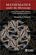Mathematics under the Microscope (Alexandre V. Borovik)

The book is saturated with amusing examples from a wide range of disciplines - from turbulence to error-correcting codes to logic - as well as with just puzzles and brainteasers.

•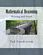Mathematical Reasoning: Writing and Proof (Ted Sundstrom)

Help students to develop logical thinking skills and to develop the ability to think more abstractly in a proof oriented setting, develop the ability to construct and write mathematical proofs using standard methods of mathematical proof including direct proofs, proof by contradiction, mathematical induction, case analysis, counterexamples.

•Category Theory for the Sciences (David I. Spivak)

Using databases as an entry to category theory, this book explains category theory by examples, and shows that category theory can be useful outside of mathematics as a rigorous, flexible, and coherent modeling language throughout the sciences.

•Mathematics and Music (David Wright)

An investigation of the interrelationships between mathematics and music, reviewing the needed background concepts in each subject as they are encountered. It explores the common foundations of the two subjects, which are developed side by side.

•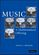Music: A Mathematical Offering (David J. Benson)

This book provides a wealth of information to understand, at varying levels of technicality, the interplay between two ancient disciplines. A must-have book if you want to know about the music of the spheres or digital music and much in between.

•How Music and Mathematics Relate (David Kung)

Understanding the connections between music and mathematics helps you appreciate both, even if you have no special ability in either field - from knowing the mathematics behind tuning an instrument to understanding the features that define your favorite pieces.

•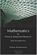Mathematics for Classical Information Retrieval: Roots and Apps

This book is about Information Retrieval (IR), particularly Classical Information Retrieval (CIR). The primary goal of book is to create a context for understanding the principles of CIR by discussing its mathematical bases.

•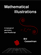Mathematical Illustrations: A Manual of Geometry and PostScript

This practical introduction to the techniques needed to produce high-quality mathematical illustrations is suitable for anyone with basic knowledge of coordinate geometry.

•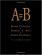A=B (Marko Petkovsek, Herbert S. Wilf, Doron Zeilberger)

This book is of interest to mathematicians and computer scientists working in finite mathematics and combinatorics. It presents a breakthrough method for analyzing complex summations.

•The Limits of Mathematics (Gregory J. Chaitin)

A course on algorithmic information theory and the epistemology of mathematics and physics. It discusses Einstein and Goedel's views on the nature of mathematics in the light of information theory, and sustains the thesis that mathematics is quasi-empirical.

•Book of Proof, 3rd Edition (Richard H. Hammack)

This book is an introduction to the language and standard proof methods of mathematics. It lays a foundation for more theoretical courses such as topology, analysis and abstract algebra.

•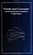Proofs and Concepts: The Fundamentals of Abstract Mathematics

This undergraduate textbook provides an introduction to proofs, logic, sets, functions, and other fundamental topics of abstract mathematics.

•Mathematics for Algorithm and Systems Analysis (E. A. Bender)

This up-to-date textbook assists undergraduates in mastering the ideas and mathematical language to address problems that arise in the field's many applications.

•An Introduction to Mathematical Reasoning (Peter J. Eccles)

The purpose of this book is to introduce the basic ideas of mathematical proof to students embarking on university mathematics.

•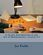A Gentle Introduction to the Art of Mathematics (David Winterfeldt)

The purpose of this book is to acclimatize the student to some of the culture and terminology of mathematics and to begin developing in them a proficiency at reading and writing mathematical proofs.

•Only Problems, not Solutions! (Florentin Smarandache)

This consists a collection of difficult mathematics problems that will challenge you to solve them.

•Magic Squares and Cubes (William Symes Andrews)

This book cover topics such as magic squares, magic cubes, the Franklin squares, magics and Pythagorean numbers, the theory of reversions, magic circles, spheres, and stars, and magic octahedroids, among other things.

•A Beautiful Math: John Nash, Game Theory, and a Code of Nature

Today neuroscientists peer into game players brains, anthropologists play games with people from primitive cultures, biologists use games to explain the evolution of human language, and mathematicians exploit games to better understand social networks.

•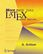More Math into LaTex: An Introduction to LaTeX and AMS-LaTeX

This is is the fourth edition of the standard introductory textbook and the complete reference of LaTeX and AMS-LaTeX for scientists in all disciplines, as well as engineers. it introduces basic ideas through sample articles and documents.

•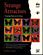Strange Attractors: Creating Patterns in Chaos (Julien C. Sprott)

Describes a simple method for generating an endless succession of beautiful fractal patterns by iterating simple maps and ordinary differential equations with coefficients chosen automatically by the computer. Each pattern produces a corresponding piece of fractal music.

•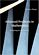Advanced Problems in Mathematics: Preparing for University

This book is intended to help candidates prepare for entrance examinations in mathematics and scientific subjects, including STEP (Sixth Term Examination Paper). is recommended as preparation for any undergraduate mathematics course.

•Quantum Computing Since Democritus (Scott Aaronson)

This book takes readers on a tour through some of the deepest ideas of maths, computer science and physics. Full of insights, arguments and philosophical perspectives, the book covers an amazing array of topics.

•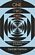One Two Three ... Infinity: Facts and Speculations of Science

Whatever your level of scientific expertise, chances are you'll derive a great deal of pleasure, stimulation, and information from this unusual and imaginative book. It belongs in the library of anyone curious about the wonders of the scientific universe.

•Logical Reasoning (Bradley H. Dowden)

The goal of this book is to improve your logical-reasoning skills. Your most important critical thinking skill is your skill at making judgments-not snap judgments that occur in the blink of an eye, but those that require careful reasoning.

Book CategoriesAll CategoriesRecent BooksMiscellaneous BooksComputer LanguagesComputer ScienceData Science/DatabasesElectrical EngineeringJava and Java EE (J2EE)Linux and UnixMathematicsMicrosoft and .NETMobile ComputingNetworking and CommunicationsSoftware EngineeringSpecial TopicsWeb Programming
Other Categories